This video was brought to you by GeneralPAC.com, making power systems Intuitive, Open and Free for Everyone, Everywhere. Consider subscribing and supporting through patreon.com/GeneralPAC. This is a mechanism for you to support us financially so we can continue making high quality power system video tutorials. Our corporate sponsor for this topic is AllumiaX.com from Seattle, Washington. Contact them for industrial and commercial power system studies.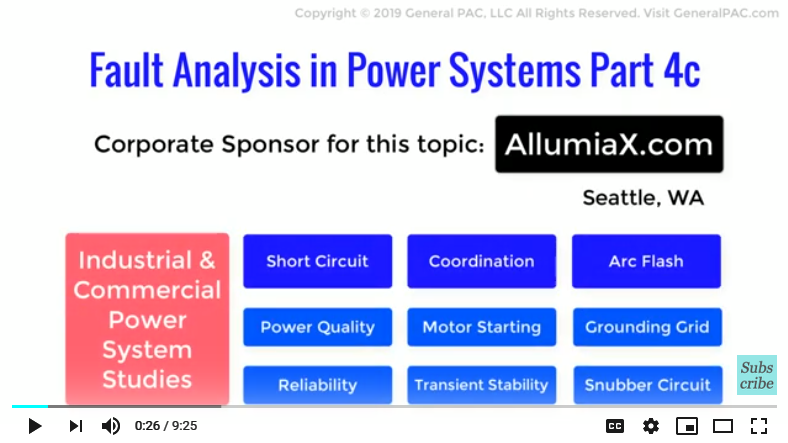Reflecting a Line to Ground Fault on HV Side, In this video we will see how we can actually reflect a 1LG fault current value which occurs on the LV side of the transformer but reflect it on the HV side.

So let’s first have a recap of our video Part 3b-1 in which we calculated fault current and voltage values for a single line to ground fault that occurs on the 13.8kV bus or the LV side of the transformer, which is shown below:

We also see the sequence network diagram as shown in that part.

For the 1LG fault type, we found that the, positive sequence current was equal to –j2.5 per unit:

And also that the positive sequence current is equal to the negative sequence current which also equals the zero sequence current and again that’s –j2.5 per unit.

That can be expressed as 2.5 angle -90 in polar form. It’s also important to note the subscript – the lower case subscript indicates fault current values on LV side. The upper case will represent fault current on the HV Side.

We now proceed by reflecting these per unit current values on the high voltage side and then we will multiply them with the appropriate base values too find the actual value.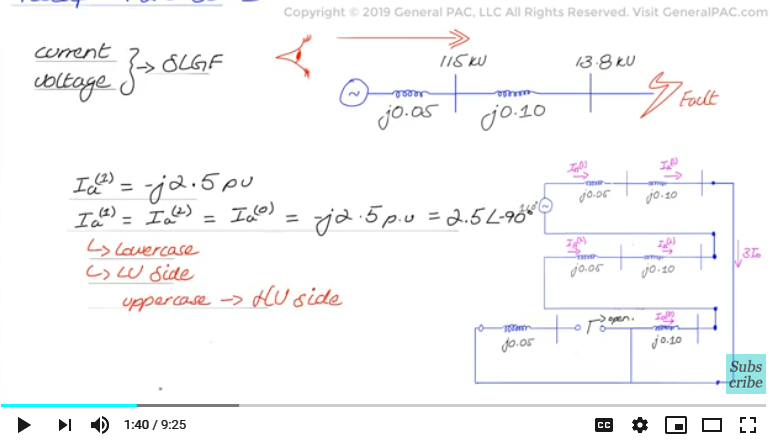So,

Given that we have a Dy1 or DAB transformer connection, to determine the HV positive sequence quantities we will shift the LV positive sequence component by +30 degrees and that can be done by multiplying the LV fault current by a value of 1 angle + 30 degrees and that gives us 2.5 at the angle of -60 degrees.

In the same way, we will shift the negative sequence component by -30 degrees, and that can be done again by multiplying the LV fault current with a value of 1 angle -30 degrees:

For the zero sequence component however, It will be nonexistent because the high voltage side is the delta side of the transformer and delta windings do not allow zero sequence currents to flow past them. They are trapped in the delta and keep flowing as circulating currents. To clarify, we again state that we will have zero seq current on the LV side, but not the HV side for this type of transformer connection.

We need to use the base values to convert the per unit quantities to ampere quantities. However, since we are working on the HV side of the transformer we divide base value of power which is 30 MVA, by the HV side voltage of 115 kV to get a base value of 150.6 Amps

And then just multiply out per units with the base value to get ampere quantities:

We get 377 Amps angle -60 degrees as the positive sequence current and 377 Amps angle -120 degrees as the negative sequence current.

Back to our familiar transformation equations, they can now be transformed to phase current quantities. Again we would remove zero sequence current from these equations since they are non-existent to simplify our calculations. These are the equations that we get

Now, plugging in the values of a to be 1 angle 120 degrees, a square to be 1 angle 240 degrees and positive and negative sequence components to be 377 angle -60 and -120 degrees respectively.

IC=(1∠120°)(377 A∠"-" 60°)+(1∠240°)(377 A∠"-" 120°)=652∠"9" 0°

We get, Line Current A equals to 625 angle -90, Line Current B = 0 and IC equals to 625 angle 90 degrees. Now these values, line up very well with current flow path on the HV side. The fault current will only flow on HV Line A and HV Line C for a LV Line A 1LG fault. The reason why the fault current will flow to line A and C on the HV side is because of the specific type of the specific Delta transformer connection we mentioned in Part 4a of this series which can also be visually seen in the diagram below:

It also makes sense that line A and C current quantities are 180 degrees apart which also supports the zero sequence calculations. Meaning, even if we tried to calculate the zero sequence current using phase A, B, and C current quantities on the HV side, well still get zero. Because I zero is equal to one third of IA + IB + IC and plugging in Line A current and Line C current we get simply equals to zero.

652 angle minus 90 degrees plus phase b current of 0 and phase c current of 652 angle 90 degree, multiplied by 1/3 gives us a 0.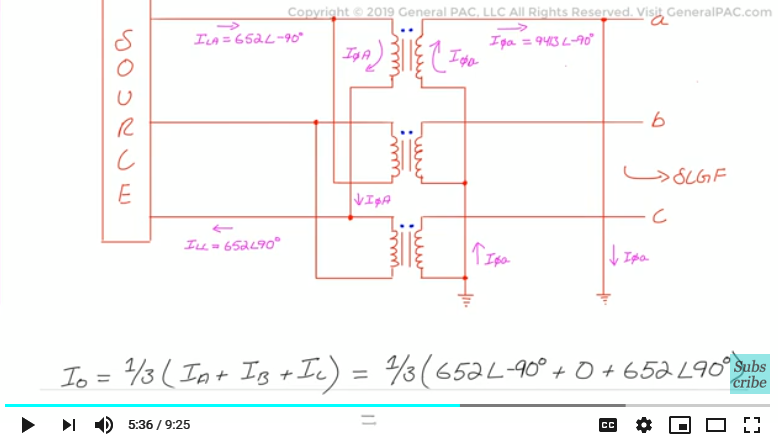Next, we will calculate the sequence voltages with our reflected sequence current components.

To do that, we go back to our faulted sequence network diagram for a single line to ground fault and apply KVL in each of the connected sequence networks.

We can calculate the positive sequence voltage by considering the voltage of the positive sequence source 1 angle 0 and the impedance of the source which is j.05 pu. The positive seq. voltage for a 1LG fault is simply the source voltage minus the voltage drop across the positive sequence impedance.

For the positive sequence voltage, we need to consider the reflected positive sequence current on the HV side, which is 2.5 angle -60 degrees. So our equation becomes, the following:

We get a value of 0.89 angle -4 degrees per unit.

Similarly, the negative sequence voltage is the source voltage minus the voltage drop across the negative sequence impedance of the high voltage side .

For the negative sequence current, we need to consider the reflected negative sequence current on the HV side which is 2.5 angle minus 120 degrees. So our equation simply becomes the following:

We get a value of 0.125 angle 150 degrees per unit.

Zero sequence voltage will be zero because the zero sequence current was also zero.

We need to set up a base value to convert per unit voltage to actual line to ground voltages. V base can therefore be taken as:

Now Multiplying the components with the base value we get the values as shown below:

The positive sequence voltage is equal to 59.3 kV angle -4 degrees and the negative sequence voltage is equal to 8.30 angle 150 kV.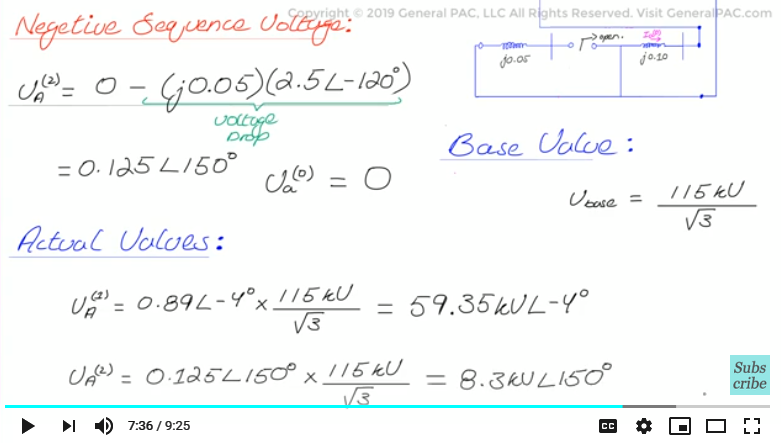Our three legacy transformation equations will then transform the sequence components to actual one line to ground phase voltages.

Again since the negative sequence component is non existent we can eliminate that directly from our equation.

Plugging in the calculated sequence component values we get the following:

We finally have the actual phase-to-ground voltages, Va is equal to 52. kV at the angle of zero degrees, Vb is equal to 66kV angle -120 degrees and Vc is 60.5 kV angle 108 degrees.

For a 1LG fault, we clearly see that the HV line A voltage is the most depressed since the fault was on line A on the LV side. We will also have some depression on HV line C voltage. We don’t see any significant depression on HV line B voltage.

This lines up very well with current flow path on the HV side. We calculated earlier that HV fault current will only flow on line A and line C for a LV 1LG fault on line a. The reason why the fault current will flow to line A and line C on the HV side is because of the specific type of Delta connection. In terms of the voltage quantities on the HV side, the largest voltage depression will occur on line A followed by line C and then line B will not see any significant depression.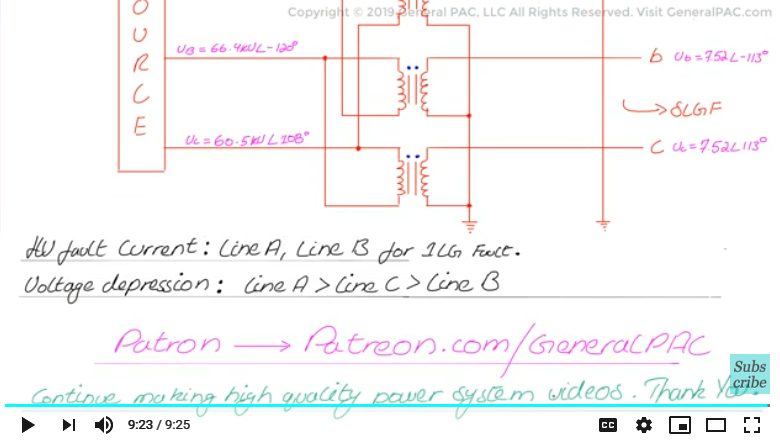Now this was a very complicated video tutorial but I hope that this video was useful and enlightening for students or professionals. Please subscribe and donate on Patreon.com/generalpac so we can continue making high quality power system video tutorials.

Our coorporate sponsor AllumiaX,LLC published a new blog on "Fault Analysis in Power Systems". This blog covers the following topics:
• Types of Faults in Power System
• Steps to perform a Fault Analysis in a Power System
• Causes of Power System Faults#### Greetings from the GeneralPAC Team!

We make high-quality Power Systems Video Tutorials on complex topics that are free and open to everyone!  Thank you so much for supporting us through Patreon so we can continue doing good and valuable work.

What is Patreon and why do we use it?

Patreon is a fantastic portal that allows our fans and community to make monthly contribution (like Netflix subscription) so we can continue creating high-quality power systems video tutorials. In return, you get access to incredible perks like voting on future topics, getting your questions answered, access to VIP Q/A webinars with the creators of GeneralPAC, and much more! We THANK YOU for supporting us

Why do we need your support?

An incredible amount of time and effort is needed to develop high-quality video tutorials. Each video (Part 1 for example) takes approximately 10 hours to complete which includes learning the concept ourselves, brainstorming creative ways to teach and explain the concepts, writing the script, audio recording, video recording, and editing. It's no wonder why Hundreds-of-Thousands of people have watched, liked, subscribed, and left positive comments on Youtube channel. Your support truly makes all the difference.